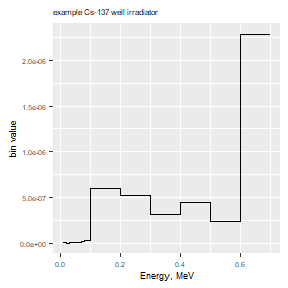library(radsafer)

The radsafer package was developed with these goals:

• Provide functions that are commonly used in radiation safety

• Share some less commonly-used functions that may be of significant value to workers in instrumentation and modeling

Related functions are identified as members of these families:

• decay corrections

• mcnp tools

This framework makes it a little easier to find the function you want - the help file for each function lists related functions.

### Decay Correction Functions

dk_correct provides decay corrected activity or activity-dependent value, such as instrument response rate or dose rate. The computation is made either based on a single radionuclide, or based on user-provided half-life, with time unit. The differential time is either computed based on dates entered or time lapsed based on the time unit.

Obtain a correction factor for a source to be used today based on a calibration on date1. Allow date2 to be the default system date.

dk_correct(half_life = 10,
time_unit = "y",
date1 = "2010-01-01")
#>  half_life RefValue    RefDate   TargDate  dk_value
#>         10        1 2010-01-01 2021-04-12 0.4576418

Use this function to correct for the value needed on dates it was used. Let the function obtain the half-life from the RadDecay data. In the example, we have a disk source with an original count rate of 10000 cpm:

dk_correct(RN_select = "Sr-90",
date1 = "2005-01-01",
date2 = c("2009-01-01","2009-10-01"),
A1 = 10000)
#>     RN half_life units decay_mode
#>  Sr-90     28.79     y         B-
#>
#>     RN RefValue    RefDate   TargDate dk_value
#>  Sr-90    10000 2005-01-01 2009-01-01 9081.895
#>  Sr-90    10000 2005-01-01 2009-10-01 8919.954

Reverse decay - find out what readings should have been in the past given today’s reading of 3000

dk_correct(RN_select = "Cs-137",
date1 = "2019-01-01",
date2 = c("2009-01-01","1999-01-01"),
A1 = 3000)
#>      RN half_life units decay_mode
#>  Cs-137   30.1671     y         B-
#>
#>      RN RefValue    RefDate   TargDate dk_value
#>  Cs-137     3000 2019-01-01 2009-01-01 3774.795
#>  Cs-137     3000 2019-01-01 1999-01-01 4749.991

Other decay functions answer the following questions:

• How long does it take to decay something with a given activity, or how old is a sample if it has decayed from? dk_time

• Given a percentage reduction in activity, how many half-lives have passed.dk_pct_to_num_half_life

• Given two or more data points, estimate the half-life: half_life_2pt

Search by alpha, beta, photon or use the general screen option.

RN_search_phot_by_E allows screening based on energy, half-life, and minimum probability. Also available are RN_search_alpha_by_E, RN_search_beta_by_E, and bin_screen_phot. RN_bin_screen_phot allows limiting searches to radionuclides with emissions in an energy bin of interest with additional filters for not having photons in other specified energy bins. Results for all these search functions may be plotted with RN_plot_spectrum.

Here’s a search for photon energy between 0.99 and 1.01 MeV, half-life between 13 and 15 minutes, and probability at least 1e-3

search_results <- RN_search_phot_by_E(0.99, 1.01, 13 * 60, 15 * 60, 1e-3)
RN code_AN E_MeV prob half_life units decay_constant
Pr-136 G 0.99100 0.0016768 13.10 m 0.0008819
Pr-136 G 1.00070 0.0503040 13.10 m 0.0008819
Re-178 G 1.00440 0.0057600 13.20 m 0.0008752
Pr-147 G 0.99597 0.0083220 13.40 m 0.0008621
Nb-88 G 0.99760 0.0041000 14.50 m 0.0007967
Mo-101 G 1.00740 0.0017300 14.61 m 0.0007907
Sm-140 G 0.99990 0.0012000 14.82 m 0.0007795
RN_plot_spectrum(search_results)
#>  "No matches"

You can also plot a spectrum in one step, skipping the data save:

 RN_plot_spectrum(
desired_RN = c("Pu-238", "Pu-239", "Am-241"), rad_type = "A",
photon = FALSE, prob_cut = 0.01, log_plot = 0)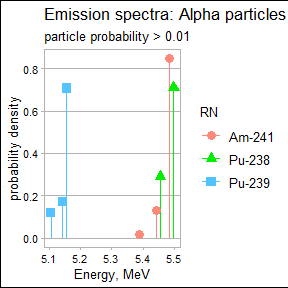The RN_index_screen function helps find a radionuclide of interest based on decay mode, half-life, and total emission energy.

In this example, we search for radionuclides decaying by spontaneous fission with half-lives between 6 months and 2 years.

RNs_selected <- RN_index_screen(dk_mode = "SF", min_half_life_seconds = 0.5 * 3.153e7, max_half_life_seconds = 2 * 3.153e7)
RN half_life units
Es-254 275.7 d
Cf-248 334.0 d

• Provide specific activity RN_Spec_Act

• Where did this radionuclide decay from? RN_find_parent

RN_find_parent("Th-230")
#>       RN
#> 1 Ac-230
#> 2 Pa-230
#> 3  U-234

air_dens_cf Correct vented ion chamber readings based on difference in air pressure (readings in degrees Celsius and mm Hg):

air_dens_cf(T.actual = 30, P.actual = 760, T.ref = 20, P.ref = 760)
#>  1.034112

Let’s try it out combined with the instrument reading:

rdg <- 100
(rdg_corrected <- rdg * air_dens_cf(T.actual = 30, P.actual = 760, T.ref = 20, P.ref = 760))
#>  103.4112

neutron_geom_cf

Correct for geometry when reading a close neutron source. Example: neutron rem detector with a radius of 11 cm and source near surface:

neutron_geom_cf(11.1, 11)
#>  0.7236467

disk_to_disk_solid_angle

Correct for a mismatch between the source calibration of a counting system and the item being measured. A significant factor in the counting efficiency is the solid angle from the source to the detector. You can also check for the impact of an item not being centered with the detector.

Example: You are counting an air sample with an active collection diameter of 45 mm, your detector has a radius of 25 mm and there is a gap between the two of 5 mm. (The function is based on radius, not diameter so be sure to divide the diameter by two.) The relative solid angle is:

(as_rel_solid_angle <- as.numeric(disk_to_disk_solid_angle(r.source = 45/2, gap = 20, r.detector = 12.5, runs = 1e4, plot.opt = "n")))
#>  0.04831014 0.00213791

An optional plot is available in 2D or 3D:

  library(ggplot2)
theme_update(# axis labels
axis.title = element_text(size = 7),
# tick labels
axis.text = element_text(size = 5),
# title
title = element_text(size = 5))
(as_rel_solid_angle <- as.numeric(disk_to_disk_solid_angle(r.source = 45/2, gap = 20, r.detector = 12.5, runs = 1e4, plot.opt = "3d")))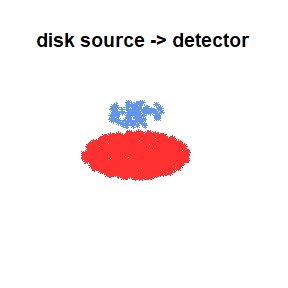#>  0.048974256 0.002164319

Continuing the example: the only calibration source you had available with the appropriate isotope has an active diameter of 20 mm. Is this a big deal? Let’s estimate the relative solid angle of the calibration, then take a ratio of the two.

(cal_rel_solid_angle <- disk_to_disk_solid_angle(r.source = 20, gap = 20, r.detector = 12.5, runs = 1e4, plot.opt = "n"))
#>    mean_eff         SEM
#>  0.05030595 0.002189261

Correct for the mismatch:

(cf <- cal_rel_solid_angle / as_rel_solid_angle)
#>  mean_eff      SEM
#>  1.027192 1.011524

This makes sense - the air sample has particles originating outside the source radius, so more of them will be lost, thus an adjustment is needed for the activity measurement.

scaler_sim

Scaler counts: obtain quick distributions for parameters of interest:

scaler_sim(true_bkg = 50, true_samp = 10, ct_time = 20, trials = 1e5)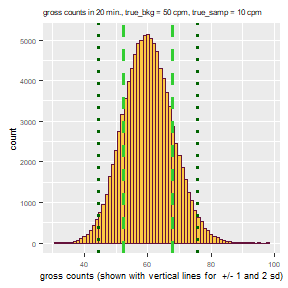rate_meter_sim

Rate meters: In the ratemeter simulation, readings are plotted once per second for a default time of 600 seconds. The meter starts with a reading of zero and builds up based on the time constant. Resolution uncertainty is established to express the uncertainty from reading an analog scale, including the instability of its readings. Many standard references identify the precision or resolution uncertainty of analog readings as half of the smallest increment. This should be considered the single coverage uncertainty for a very stable reading. When a reading is not very stable, evaluation of the reading fluctuation is evaluated in terms of numbers of scale increments covered by meter indication over a reasonable evaluation period. Example with default time constant:

rate_meter_sim(cpm_equilibrium = 270, meter_scale_increments = seq(100, 1000, 20))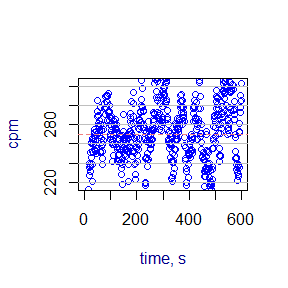To estimate time constant, use tau.estimate

### Stay-time computation

Given a dose rate, dose allowed, and a safety margin (default = 20%), calculate stay time with: stay_time

stay_time(dose_rate = 120, dose_allowed = 100, margin =  20)
#>  "Time allowed is 40 minutes"
#>  40

### mcnp tools functions

If you create MCNP inputs, these functions may be helpful:

mcnp_si_sp_RD Obtain emission data from the RadData package and write to a file for use with the radiation transport code, MCNP.

mcnp_si_sp_hist

• Create an energy distribution from histogram data with: mcnp_si_sp_hist Or use mcnp_si_sp_hist_scan to quickly copy and paste data interactively.

mcnp_matrix_rotations

• Determine the entries needed for MCNP coordinate transformation rotation

mcnp_cone_angle

• Quickly obtain the cone angle entry

mcnp_plot_out_spec

For MCNP outputs, plot the results of a tally with energy bins. The fastest way to do this is with mcnp_scan2plot.

Alternatively, if you want to get your data into R, first save it in a text file and import it to R. (Base R provides methods with read.table or you might prefer options from the readr or readxl packages.) Or you can copy and paste your data using mcnp_scan_save. You can then plot with mcnp_plot_out_spec (below) or design your own plot.

mcnp_plot_out_spec(photons_cs137_hist, 'example Cs-137 well irradiator')